## Maths

#### Computational Physics Basics: Accuracy and Precision

Problems in physics almost always require us to solve mathematical equations with real-valued solutions, and more often than not we want to find functional dependencies of some quantity of a real-valued domain. Numerical solutions to these problems will only ever be approximations to the exact solutions. When a numerical outcome of the calculation is obtained it is important to be able to quantify to what extent it represents the answer that was sought. Two measures of quality are often used to describe numerical solutions: accuracy and precision. Accuracy tells us how will a result agrees with the true value and precision tells us how reproducible the result is. In the standard use of these terms, accuracy and precision are independent of each other.

#### Accuracy

Accuracy refers to the degree to which the outcome of a calculation or measurement agrees with the true value. The technical definition of accuracy can be a little confusing because it is somewhat different from the everyday use of the word. Consider a measurement that can be carried out many times. A high accuracy implies that, on average, the measured value will be close to the true value. It does not mean that each individual measurement is near the true value. There can be a lot of spread in the measurements. But if we only perform the measurement often enough, we can obtain a reliable outcome.

#### Precision

Precision refers to the degree to which multiple measurements agree with each other. The term precision in this sense is orthogonal to the notion of accuracy. When carrying out a measurement many times high precision implies that the outcomes will have a small spread. The measurements will be reliable in the sense that they are similar. But they don’t necessarily have to reflect the true value of whatever is being measured.

#### Accuracy vs Precision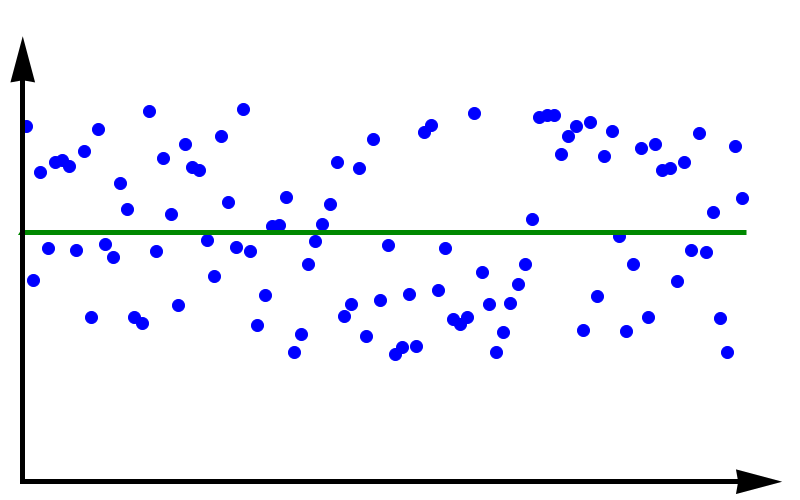Data with high accuracy but low precision. The green line represents the true value.

To fully grasp the concept of accuracy vs precision it is helpful to look at these two plots. The crosses represent measurements whereas the line represents the true value. In the plot above, the measurements are spread out but they all lie around the true value. These measurements can be said to have low precision but high accuracy. In the plot below, all measurements agree with each other, but they do not represent the true value. In this case, we have high precision but low accuracy.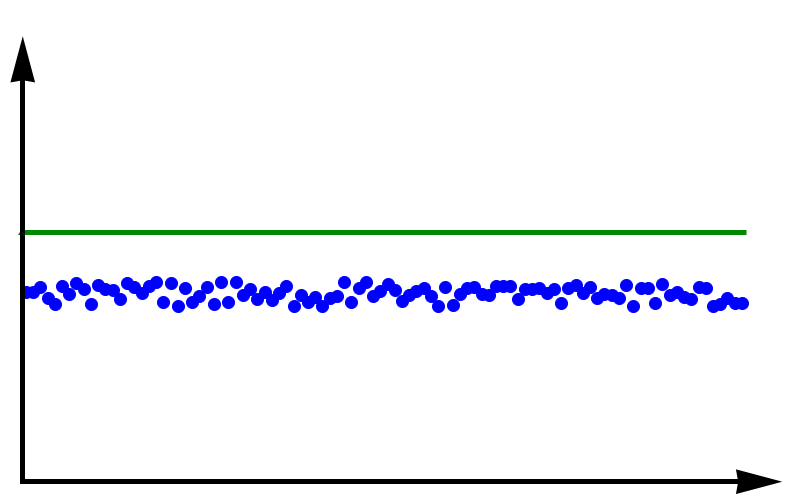Data with high precision but low accuracy. The green line represents the true value.

A moral can be gained from this: just because you always get the same answer doesn’t mean the answer is correct.

When thinking about numerical methods you might object that calculations are deterministic. Therefore the outcome of repeating a calculation will always be the same. But there is a large class of algorithms that are not quite so deterministic. They might depend on an initial guess or even explicitly on some sequence of pseudo-random numbers. In these cases, repeating the calculation with a different guess or random sequence will lead to a different result.

#### What are Numbers? Or, Learning to Count!

We all use numbers every day and some of us feel more comfortable dealing with them than others. But have you ever asked yourself what numbers really are? For example, what is the number 4? Of course, you can describe the symbol “4”. But that is not really the number, is it? You can use Roman numerals IV, Urdu ۴, or Chinese and Japanese Kanji . Each one of these symbols represents the same number. And yet, somehow we would all probably agree that there is only one number 4.

The question about the nature of numbers is twofold. You can understand this question purely mathematical one and ask for a clear definition of a number and the set of numbers in a mathematical sense. This will be the topic of this article. You can also ask yourself what numbers are in a philosophical sense. Do numbers exist? If yes, in what way do they exist, and what are they? This may be the topic of a future article.

Now that we have settled what type of question we want to answer, we should start with the simplest type of numbers. These are the natural numbers 0, 1, 2, 3, 4, …
I decided to include the number zero even though it might seem a little abstract at first. After all, what does it mean to have zero of something? But that objection strays into the philosophical realm again and, as I said above, I want to focus on the mathematical aspect here.

When doing the research for this article, I was slightly surprised at the plethora of different definitions for the natural numbers. But given how fundamental this question is, it should be no wonder that many mathematicians have thought about the problem of defining numbers and have come up with different answers.

## The Peano Axioms

Let’s start with an axiomatic definition of numbers called the Peano axioms. This is one of the earliest strict definitions of the natural numbers in the modern sense. It doesn’t really state what the natural numbers are but focuses on how they behave. We start with the set of natural numbers, which we call $\mathbb{N}$, and a successor function $S$.

Peano Axiom 1:

$0$ is a natural number or, more formally, $0 \in \mathbb{N}$

This axiom just tells us that there is a natural number zero. We could have chosen 1 as the starting point but this is arbitrary.

Peano Axiom 2:

Every natural number $x$ has a successor $y$.
In other words, given that $x \in \mathbb{N}$ then it follows that $y = S(x) \in \mathbb{N}$.

Intuitively, the successor function will always produce the next natural number.
Mathematicians call this property of the natural number being closed under the successor operation $S$. All this means is that the successor operation will never produce a result that is outside of the natural numbers.

Peano Axiom 3:

If we have two natural numbers $x$ and $y$, and we know that the successors of $x$ and $y$ are equal, then this is the same as saying that $x$ and $y$ are equal.
Again, written more formally we say, given $x \in \mathbb{N}$ and $y \in \mathbb{N}$ and $S(x) = S(y)$ then it follows that $x=y$.

This means that any natural number cannot be the successor of two different number. In other words, if you have two different numbers then they can’t have the same successor.

Peano Axiom 4:

$0$ is not the successor of any other natural number.
In mathematical notation, if $x \in \mathbb{N}$ then $S(x) \ne 0$

At first sight, we might think that these axioms are complete. We can start from zero and use the successor function $S$ to iterate through the natural numbers. This is intuitively shown in the image below.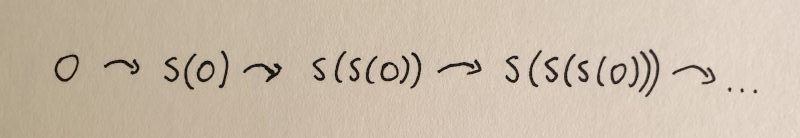Repeatedly applying the successor function, starting from 0.

Here
1 = S(0)
2 = S(S(0)) = S(1)
3 = S(S(S(0))) = S(2)
and so on.

But we haven’t guaranteed that this iteration will eventually be able to reach all natural numbers. Imagine that, in addition to the above, there is some special number $z$ that creates a closed loop when applying the successor function. And this loop is separate from the sequence that we get when starting from zero.A closed loop of successors that isn’t connected to the zero element.

So we need another axiom that guarantees that all natural numbers are reachable from zero by repeatedly applying the successor function. This axiom is usually stated in the following way.

Peano Axiom 5: Axiom of Induction

Given any set $U \subseteq \mathbb{N}$ with $0 \in U$.
If $U$ is such that for every $n \in U$ the successor is also in $U$, i.e. $S(n) \in U$,
then $U = \mathbb{N}$

The idea behind this axiom is that $U$ can be chosen as the minimal set that contains no additional loops or numbers that are not reachable from zero. The fact that any set $U$ that contains zero and is closed under the successor function is identical to the natural numbers guarantees that all natural numbers are eventually reachable from zero. The axiom of induction is maybe more familiar in its alternative form.

Peano Axiom 5: Axiom of Induction, alternative form

Consider a mathematical statement that is true for zero.
If it can be proven that,
given the statement is true for a number $n$, then it is also true for $S(n)$,
then it follows that the statement is true for all natural numbers.

Here you can see that this axiom forms the basis of the familiar proof by induction.

## Some Remarks

The Peano Axioms are helpful in defining the set of natural numbers $\mathbb{N}$ and arithmetic operations on them. But personally, I feel unsatisfied by this definition. The Peano Axioms tell us how natural numbers behave but they don’t really give any additional insight as to what numbers really are.

Take for example the number 2. We now know that 2 can be expressed as the successor of the successor of 0, i.e. $2 = S(S(0))$. We also know that this second successor must be a member of the set of natural numbers, but not much more. The problem here is that we never defined what the successor function should be.

Nonetheless, the Peano axioms can serve as a basis for more in-depth definitions of the natural numbers. These definitions can be considered models of the Peano Axioms in the sense that they define a zero element and some concrete successor function. The set of natural numbers can then be constructed from these and the Peano Axioms follow as consequences from these definitions.

In a future post, I will look at some set-theoretic definitions of the natural numbers. If you liked this post, please leave a comment and check back soon for more content.

#### The Euler Equations: Sod Shock Tube

In the last post, I presented a simple derivation of the Euler fluid equations. These equations describe hydrodynamic flow in the form of three conservation equations. The three partial differential equations express the conservation of mass, the conservation of momentum, and the conservation of energy. These fundamental conservation equations are written in terms of fluxes of the densities of the conserved quantities.

In general, it is impossible to solve the Euler equations for an arbitrary problem. This means that in practice when we want to find the hydrodynamic flow in a particular situation, we have to use computers and numerical methods to calculate an approximation to the solution.

Numerical simulation codes approximate the continuous mathematical solution by storing the values of the functions at discrete points. The values are stored using a finite precision format. On most CPUs nowadays numbers will be stored as 64-bit floating-point, but for codes running on GPUs, this might be reduced to 32 bits. In addition to the discretisation in space, the equations are normally integrated using discrete steps in time. All of these factors mean that the computer only stores part of the information of the exact function.

These discretisations mean that the continuous differential equations have to be turned into a prescription how to update the discrete values from one time step to the next. For each differential equation, there are numerous ways to translate them into a numerical algorithm. Each algorithm will make different approximations and introduce different errors into the system. In general, it is impossible for a numerical algorithm to reproduce the solutions of the mathematical equations exactly. This will have implications for the physics that will be simulated with the code. Talking in terms of the Euler equations, some numerical algorithms will have problems capturing shocks, while others might smear out the solutions. Even others might cause the density to turn negative in places. Making sure that physical invariants, such as mass, momentum, and energy are conserved by the algorithm is often an important aspect when designing a numerical scheme.

### The Sod Shock Tube

One of the standard tests for numerical schemes simulating the Euler equations is the Sod shock tube problem. This is a simple one-dimensional setup that is initialised with a single discontinuity in the density and energy density. It then develops a left-going rarefaction wave, a right-going shock and a somewhat slower right-going contact discontinuity. The shock tube has been proposed as a test for numerical schemes by Gary A. Sod 1978 . It was then picked up by others like Philip Roe  or Bram van Leer  who made it popular. Now, it is one of the standard tests for any new solver for the Euler equations. The advantage of the Sod Shock Tube is that it has an analytical solution that can be compared against. I won’t go into the details of deriving the solution in this post. You can find a sketch of the procedure on Wikipedia.
Here, I want to present an example of the shock tube test using an algorithm by Kurganov, Noelle, and Petrova . The advantage of the algorithm is that it is relatively easy to implement and that is straightforward to extend to multiple dimensions. I have implemented the algorithm in my open-source fluid code Vellamo. At the current stage, the code can only simulate the Euler equations, but it can run in 1d, 2d, or 3d. It is also parallelised so that it can run on computer clusters It uses the Schnek library to manage the computational grids, their distribution onto multiple CPUs, and the communication between the individual processes.

The video shows four simulations run with different grid resolutions. At the highest resolution of 10000 grid points, the results are more or less identical to the analytic solution. The left three panels show the density $\rho$, the momentum density $\rho v$, and the energy density $E$. These are the quantities that are actually simulated by the code. The right three panels show quantities derived from the simulation, the temperature $T$, the velocity $v$, and the pressure $p$.

Starting from the initial discontinuity at $x=0.5$ you can see a rarefaction wave moving to the left. The density $\rho$ has a negative slope whereas the momentum density $\rho v$ increases in this region. In the plot of the velocity, you can see a linear slope, indicating that the fluid is being accelerated by the pressure gradient. The expansion of the fluid causes cooling and the temperature $T$ decreases inside the rarefaction wave.

While the rarefaction wave moves left, a shock wave develops on the very right moving into the undisturbed fluid. All six graphs show a discontinuity at the shock. The density jumps $\rho$ as the fluid is compressed and the velocity $v$ rises from 0 to almost 1 as the expanding fluid pushes into the undisturbed background. The compression also causes a sudden increase in pressure $p$ and temperature $T$.

To the left of the shock wave, you can observe another discontinuity in some but not all quantities. This is what is known as a contact discontinuity. To the left of the discontinuity, the density $\rho$ is high and the temperature $T$ is low whereas to the right density $\rho$ is low and the temperature $T$ is high. Both effects cancel each other when calculating the pressure which is the same on both sides of the discontinuity. And because there is no pressure difference, the fluid is not accelerated either, and the velocity $v$ is the same on both sides as well.

If you look at the plots of the velocity $v$ and the pressure $p$ you can’t make out where the contact discontinuity is. In a way, this is quite extraordinary because these two quantities are calculated from the density $\rho$, the momentum density $\rho v$ and the energy density $E$. All of these quantities have jumps at the location of the contact discontinuity. The level to which the derived quantities stay constant across the discontinuity is an indicator of the quality of the numerical scheme.

The simulations with lower grid resolutions show how the scheme degrades. At 1000 grid points, the results are still very close to the exact solution. The discontinuities are slightly smeared out. If you look closely at the temperature profile, you can see a slight overshoot on the high-temperature side of the contact discontinuity. At 100 grid points, the discontinuities are more smeared out. The overshoot in the temperature is more pronounced and now you can also see an overshoot at the right end of the rarefaction wave. This overshoot is present in most quantities.

As a rule of thumb, a numerical scheme for hyperbolic differential equations has to balance accuracy against numerical diffusion. In regions where the solution is well behaved, high order schemes will provide very good accuracy. Near discontinuities, however, a high order scheme will tend to produce artificial oscillations. These artefacts can create non-physical behaviour when, for instance, the numerical solution predicts a negative density or temperature. A good scheme detects the conditions where the high order algorithm fails and falls back to a lower order in those regions. This will naturally introduce some numerical diffusion into the system.

At the lowest resolution with 50 grid points, the discontinuities are even more spread out. Nonetheless, at later times in the simulation, all discontinuities can be seen and the speed at which these discontinuities move compares well with the exact result.

 Sod, G.A., 1978. A survey of several finite difference methods for systems of nonlinear hyperbolic conservation laws. Journal of computational physics, 27(1), pp.1-31.
 Roe, P.L., 1981. Approximate Riemann solvers, parameter vectors, and difference schemes. Journal of computational physics, 43(2), pp.357-372.
 Van Leer, B., 1979. Towards the ultimate conservative difference scheme. V. A second-order sequel to Godunov’s method. Journal of Computational Physics, 32(1), pp.101-136.
 Kurganov, A., Noelle, S. and Petrova, G., 2001. Semidiscrete central-upwind schemes for hyperbolic conservation laws and Hamilton–Jacobi equations. SIAM Journal on Scientific Computing, 23(3), pp.707-740.

#### The Euler Equations

Leonhard Euler was not only brilliant but also a very productive mathematician. In this post, I want to talk about one of the many things named after the Swiss genius, the Euler equations. These should not be confused with the Euler identity, that famous relation involving $e$, $i$, and $\pi$. No, the Euler equations are a set of partial differential equations that can be used to describe fluid flow. They are related to the Navier-Stokes equations which are at the heart of one of the unsolved Millenium Problems of the Clay Mathematics Institute.

In this short series of posts, I want to present a phenomenological derivation of the equations in one dimension. This derivation is not mathematically rigorous, but will hopefully give you an understanding of the physics that the equations are describing.

### Conservation Laws

One way to understand the Euler equations is to write them in the form of conservation laws. Each equation represents a basic conservation law of physics. A central concept in these conservation equation is flux. In a 1d description, the flux of a quantity is a measure for the amount of that quantity that passes through a point. In 2d it’s the amount passing through a line and in 3d through a surface, but let’s stick to 1d during this article.

Take some quantity $N$. To make it concrete, imagine something like the total mass or number of atoms in a volume. I am using the word volume loosely. In 1d a volume is the same as an interval. Let’s call the flux of this quantity $F$. The flux can be different at different locations, so $F$ is a function of position $x$, in other words, the flux is $F(x)$.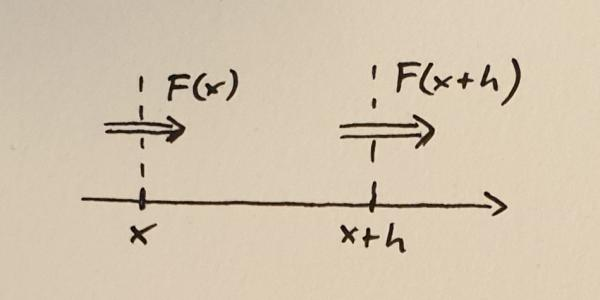Flux into and out of a small volume between $x$ and $x+h$

Next, consider a small volume between $x$ and $x+h$. Here $h$ is the width of the volume and you should think of it as a small quantity. The rate of change of the quantity $N$ within this volume is determined by the difference between the flux into the volume and the flux out of the volume. If we take positive flux to mean that the quantity (mass, number of atoms, stuff) is moving to the right, then we can write down the following equation.

$$\frac{dN}{dt} = F(x) – F(x+h)$$

Here the left-hand side of the equation represents the rate of change of $N$. I have not written it here but, of course, $N$ depends on the position $x$ as well as the with of the volume $h$. The next step to turn this into a conservation equation is to divide both sides by $h$.

$$\frac{d}{dt}\left(\frac{N}{h}\right) = -\frac{F(x+h) – F(x)}{h}$$

If you remember your calculus lessons, you might already see where this is going. We now make $h$ smaller and smaller and look at the limit when $h \to 0$. The right hand side of the equation will give us the derivative of $F$,
$$\lim_{h \to 0} \frac{F(x+h) – F(x)}{h} = \frac{dF}{dx}$$

The amount of stuff $N$ in the volume will shrink and shrink as $h$ decreases, but because we are dividing by $h$ the ratio $N/h$ will converge to a sensible value. Let’s call this value $n$,

$$n = \lim_{h \to 0}\frac{N}{h}.$$

The quantity $n$ is called the density of $N$. Now the conservation law is simply written as

$$\frac{\partial n}{\partial t} = -\frac{\partial F}{\partial x}.$$

This equation expresses the change of the density of the conserved quantity through the derivative of the flux of that quantity.

### The Equations

Now that the basic form of the conservation equations is established, let’s look at some quantities that are conserved and that can be used to express fluid flow. The first conservation law to look at is the conservation of mass. Note again that the conservation equation is written in terms of the density of the conserved quantity. The mass density is the mass contained in a small volume divided by that volume. It is often abbreviated by the Greek letter $\rho$ (rho).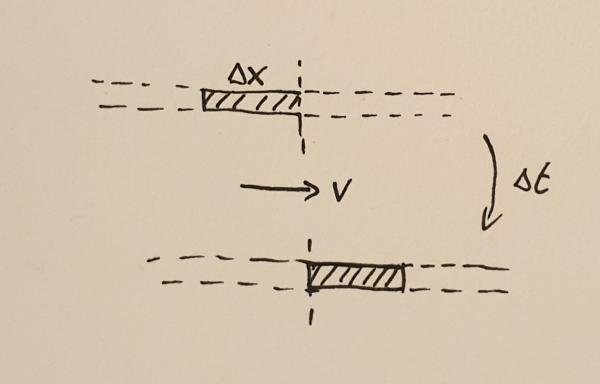Matter crossing a point during the time interval $\Delta t$

In order to find out what the mass flux is, consider the fluid moving with velocity $v$ through some point. During a small time interval, $\Delta t$ the fluid will move by a small distance $\Delta x$. All the mass $M$ contained in the interval of width $\Delta x$ will cross the point. This mass can be calculated to be

$$M = \rho \Delta x.$$

The flux is the amount of mass crossing the point divided by the time it took the mass to cross that point, $F_{\rho} = M / \Delta t$. In other words,

$$F_{\rho} = \rho \frac{\Delta x}{\Delta t} = \rho v.$$

This now lets us write the mass conservation equation,

$$\frac{\partial \rho}{\partial t} = -\frac{\partial }{\partial x}(\rho v).$$

What does this equation mean? Let me explain this with two examples. First, imagine a constant flow velocity $v$ but an increasing density profile $\rho(x)$. At any given point the density will decrease because the density profile will constantly move towards the right without changing shape. The lower density that was previously located at somewhat left of $x$ will move to the point $x$ thus decreasing the density there.

For the other example, imagine that the density is constant but the velocity has an increasing profile. Matter to the right will flow faster than matter to the left. This will also decrease the density over time because the existing mass is constantly thinned out.

The next conservation equation expresses the conservation of momentum. Momentum is mass times velocity and therefore momentum density is mass density times velocity, $\rho v$. In the mass conservation equation, you could see that the flux consists only of the conserved density multiplied with the velocity. This is called the convective term and this term is present in all Euler equations. However, the momentum can also change through a force. Without external forces, the only force onto each fluid element is through the pressure $p$. The momentum conservation equation can be written as

$$\frac{\partial }{\partial t}(\rho v) = -\frac{\partial }{\partial x}(\rho v^2 + p).$$

The last conservation equation is the conservation of energy, expressed by the energy density $e$,

$$\frac{\partial e}{\partial t} = -\frac{\partial }{\partial x}(e v + p v).$$

The first term is again the convective term expressing the transport of energy contained in the fluid as the fluid moves. The second term is the work done by the pressure force. Note that the energy density $e$ contains the internal (heat) energy as well as the kinetic energy of the fluid moving as a whole.

Finally, we need to close the set of equations by defining what the pressure $p$ is. This closure depends on the type of fluid you want to model. For an ideal gas, the pressure is related to the other variables through

$$p = (\gamma – 1)\left(E – \frac{\rho v^2}{2}\right).$$

Here $\gamma$ is the adiabatic gas index. The second bracket on the right-hand side is the internal energy, expressed as the total energy minus the kinetic energy of the flow.

### Putting it All Together

In summary, the Euler equations are conservation equations for the mass, momentum, and energy in the system. In 1d, they can be written as

$$\begin{eqnarray} \frac{\partial }{\partial t}\rho &=& -\frac{\partial }{\partial x}(\rho v) \\ \frac{\partial }{\partial t}(\rho v) &=& -\frac{\partial }{\partial x}(\rho v^2 + p) \\ \frac{\partial }{\partial t}e &=& -\frac{\partial }{\partial x}(e v + p v) \end{eqnarray}$$

In higher dimensions, the derivative with respect to $x$ is replaced by the divergence operator $\nabla$.

$$\begin{eqnarray} \frac{\partial }{\partial t}\rho &=& -\nabla(\rho \mathbf{v}) \\ \frac{\partial }{\partial t}(\rho v) &=& -\nabla(\rho \mathbf{v}\otimes\mathbf{v} + p\mathbf{I}) \\ \frac{\partial }{\partial t}e &=& -\nabla(e \mathbf{v} + p \mathbf{v}) \end{eqnarray}$$

The $\otimes$ symbol denotes the outer product of the velocity vectors. It multiplies two vectors to produce a matrix. I will not go into the definition of the outer product here. You can read more on Wikipedia. The symbol $\mathbf{I}$ is the identity matrix.

### Summary

The Euler equations are a set of partial differential equations describing fluid flow. When Euler published a simpler version of these equations, they were one of the earliest examples of partial differential equations. Today, the Euler equations and their generalisations, including the Navier-Stokes equations provide the basis for understanding fluid behaviour, turbulence, and the weather.

The Euler equations are difficult to solve and only some special solutions can be written down on paper. For the general case, we need computers to give us answers. In the next post, I will show the results of some simulations I have created with my freely available fluid code Vellamo.

#### Computational Physics Basics: Integers in C++, Python, and JavaScript

In a previous post, I wrote about the way that the computer stores and processes integers. This description referred to the basic architecture of the processor. In this post, I want to talk about how different programming languages present integers to the developer. Programming languages add a layer of abstraction and in different languages that abstraction may be less or more pronounced. The languages I will be considering here are C++, Python, and JavaScript.

### Integers in C++

C++ is a language that is very close to the machine architecture compared to other, more modern languages. The data that C++ operates on is stored in the machine’s memory and C++ has direct access to this memory. This means that the C++ integer types are exact representations of the integer types determined by the processor architecture.

The following integer datatypes exist in C++

Type Alternative Names Number of Bits G++ on Intel 64 bit (default)
char at least 8 8
short int short at least 16 16
int at least 16 32
long int long at least 32 64
long long int long long at least 64 64

This table does not give the exact size of the datatypes because the C++ standard does not specify the sizes but only lower limits. It is also required that the larger types must not use fewer bits than the smaller types. The exact number of bits used is up to the compiler and may also be changed by compiler options. To find out more about the regular integer types you can look at this reference page.

The reason for not specifying exact sizes for datatypes is the fact that C++ code will be compiled down to machine code. If you compile your code on a 16 bit processor the plain int type will naturally be limited to 16 bits. On a 64 bit processor on the other hand, it would not make sense to have this limitation.

Each of these datatypes is signed by default. It is possible to add the signed qualifier before the type name to make it clear that a signed type is being used. The unsigned qualifier creates an unsigned variant of any of the types. Here are some examples of variable declarations.

char c; // typically 8 bit
unsigned int i = 42; // an unsigned integer initialised to 42
signed long l; // the same as "long l" or "long int l"


As stated above, the C++ standard does not specify the exact size of the integer types. This can cause bugs when developing code that should be run on different architectures or compiled with different compilers. To overcome these problems, the C++ standard library defines a number of integer types that have a guaranteed size. The table below gives an overview of these types.

Signed Type Unsigned Type Number of Bits
int8_t uint8_t 8
int16_t uint16_t 16
int32_t uint32_t 32
int64_t uint64_t 64

More details on these and similar types can be found here.

The code below prints a 64 bit int64_t using the binary notation. As the name suggests, the bitset class interprets the memory of the data passed to it as a bitset. The bitset can be written into an output stream and will show up as binary data.

#include  <bitset>

void printBinaryLong(int64_t num) {
std::cout << std::bitset<64>(num) << std::endl;
}


### Integers in Python

Unlike C++, Python hides the underlying architecture of the machine. In order to discuss integers in Python, we first have to make clear which version of Python we are talking about. Python 2 and Python 3 handle integers in a different way. The Python interpreter itself is written in C which can be regarded in many ways as a subset of C++. In Python 2, the integer type was a direct reflection of the long int type in C. This meant that integers could be either 32 or 64 bit, depending on which machine a program was running on.

This machine dependence was considered bad design and was replaced be a more machine independent datatype in Python 3. Python 3 integers are quite complex data structures that allow storage of arbitrary size numbers but also contain optimizations for smaller numbers.

It is not strictly necessary to understand how Python 3 integers are stored internally to work with Python but in some cases it can be useful to have knowledge about the underlying complexities that are involved. For a small range of integers, ranging from -5 to 256, integer objects are pre-allocated. This means that, an assignment such as

n = 25

will not create the number 25 in memory. Instead, the variable n is made to reference a pre-allocated piece of memory that already contained the number 25. Consider now a statement that might appear at some other place in the program.

a = 12
b = a + 13

The value of b is clearly 25 but this number is not stored separately. After these lines b will reference the exact same memory address that n was referencing earlier. For numbers outside this range, Python 3 will allocate memory for each integer variable separately.

Larger integers are stored in arbitrary length arrays of the C int type. This type can be either 16 or 32 bits long but Python only uses either 15 or 30 bits of each of these "digits". In the following, 32 bit ints are assumed but everything can be easily translated to 16 bit.

Numbers between −(230 − 1) and 230 − 1 are stored in a single int. Negative numbers are not stored as two’s complement. Instead the sign of the number is stored separately. All mathematical operations on numbers in this range can be carried out in the same way as on regular machine integers. For larger numbers, multiple 30 bit digits are needed. Mathamatical operations on these large integers operate digit by digit. In this case, the unused bits in each digit come in handy as carry values.

### Integers in JavaScript

Compared to most other high level languages JavaScript stands out in how it deals with integers. At a low level, JavaScript does not store integers at all. Instead, it stores all numbers in floating point format. I will discuss the details of the floating point format in a future post. When using a number in an integer context, JavaScript allows exact integer representation of a number up to 53 bit integer. Any integer larger than 53 bits will suffer from rounding errors because of its internal representation.

const a = 25;
const b = a / 2;

In this example, a will have a value of 25. Unlike C++, JavaScript does not perform integer divisions. This means the value stored in b will be 12.5.

JavaScript allows bitwise operations only on 32 bit integers. When a bitwise operation is performed on a number JavaScript first converts the floating point number to a 32 bit signed integer using two’s complement. The result of the operation is subsequently converted back to a floating point format before being stored.

#### The SIR Model for the Spread of Infectious Diseases

In the current Coronavirus crisis, everybody is talking about flattening “the curve”. In the news, you will often see graphs of the total number of cases or the total number of deaths over time. So you may be forgiven to think that these are the curves that everybody is trying to flatten. In fact, what epidemiologists mean by the curve is the graph of the number of actively infected people over time. This curve is important because it determines the load that is placed on the healthcare system of a country. The current number of cases determines how many hospital beds, how many ventilators, and how much healthcare personnel are needed.

Mathematics and computer simulations play an important role in estimating how the disease will spread, how many people will be affected, and how much resources are needed. They also allow predicting the effects of different measures to control the spread. For example, the current lockdown in many countries around the world is reducing the number of people that an infected individual can pass the virus on to. It is important to know how effective this measure is. One of the big questions is when it is safe to relax the isolation of people and how much it would affect the spread if individual businesses re-open.

Before continuing, I have to add a disclaimer. I am interested in mathematics but I am not an expert epidemiologist. The models I am showing you here are very simple starting points for simulating the spread of diseases. They can give you some idea on how parameters like the infection rate and recovery rate influence the overall number of infected individuals. But they should not be used to draw any quantitative conclusions.

#### The SIR Model

To get a basic feel for the way infections spread through a population, epidemiologists have developed simple mathematical models. Probably the first model you will hear about in this context is the SIR model. The SIR model is a version of a compartmental model. This means that the total population is divided up into separate compartments. The quantity $S$ denotes the number of susceptible individuals. These are the people that are not infected and also don’t have any immunity to the disease. $I$ is the number of infected individuals and $R$ is the number of individuals that are not infectious but also can’t get the disease. Most scientists denote the $R$ to mean removed as it includes both people who have recovered and are immune but also those that have died. Due to the current sensitivity of the subject, many people prefer to call $R$ the recovered population.

Compartmental models define rates by which individuals change from one population to another. The SIR model has two rates, the rate of infection and the rate of recovery. The absolute rate of infection is proportional to the number of infected people. On average, each infected individual will pass the infection to a number of people in a given time interval. This number is usually called $\beta$. However, if an infected individual passes the virus to a recovered person, the infection will not spread. The probability of passing the infection on is given by $S/N$ where $N$ is the total population $N=S+I+R$. Putting this together, the absolute rate of infection is

$$\frac{\beta I S}{N}.$$

The rate of recovery is slightly more simple. Each infected individual will recover with some probability $\gamma$ in a given time interval. The absolute rate of recovery is then expressed as

$$\gamma I.$$
The infection rate reduces the number of susceptible individuals $S$ and increases the number of infected individuals $I$. The recovery rate reduces the number of infected individuals $I$ and increases the number of recovered individuals $R$. The complete set of rate equations is then

$$\begin{eqnarray} \frac{dS}{dt} &=& – \frac{\beta I S}{N}, \\ \frac{dI}{dt} &=& \frac{\beta I S}{N} – \gamma I, \\ \frac{dR}{dt} &=& \gamma I. \end{eqnarray}$$

The ratio of the coefficients $\beta$ and $\gamma$ is known as the basic reproduction ratio.

$$R_0 = \frac{\beta}{\gamma}$$.

The $R_0$ is important because it determines whether the infection will spread exponentially or eventually die out.

I have implemented a little JavaScript app that integrates the SIR equations and shows the development of the populations over time. Feel free to play around with the sliders and explore how the parameters influence the spread.

I encourage you to play around with the parameters to see how the model behaves. For an infection rate of 1 and a recovery rate of 0.5, the populations stabilise when about 80% of the population has been infected and has recovered. The maximum of the infectious population, the $I$ curve, reaches about 16%. If you reduce the infection rate, the $I$ curve flattens, prolonging the time over which the disease is spreading but reducing the maximum number of infected individuals at any one time.

#### The SEIR Model

One of the major assumptions in the SIR model is that an infected individual can immediately spread the infection. A refinement of the model is the addition of a population, $E$, of exposed individuals. These are people that are infected but are not yet infectious. The SEIR model introduces another rate, $a$, at which exposed individuals turn infectious. The quantity $a$ can be understood as the inverse of the average incubation period. The absolute rate at which exposed individuals become infectious is

$$a E.$$

The complete set of equations of the SEIR model are then as follows.

$$\begin{eqnarray} \frac{dS}{dt} &=& – \frac{\beta I S}{N}, \\ \frac{dE}{dt} &=& \frac{\beta I S}{N} – a E, \\ \frac{dI}{dt} &=& a E – \gamma I, \\ \frac{dR}{dt} &=& \gamma I. \end{eqnarray}$$

The SEIR model is also implemented in the app above. Simply pick SEIR Model from the dropdown menu and start exploring.

#### The SEIR Model with Delay

The SEIR model above assumes that an individual, once exposed, can immediately turn infectious. The constant rate $a$ implies that the probability of changing from the exposed state to the infectious state is the same on day one of being exposed as it is on day ten. This might not be realistic because diseases typically have some incubation period. Only after some number of days after being exposed will an individual become infectious. One can model this kind of behaviour with a time delay. Let’s say that after a given incubation period $\tau$, every exposed individual will turn infectious. The absolute rate at which exposed individuals become infectious is then given by

$$\frac{\beta I(t-\tau) S(t-\tau)}{N}.$$

Here the $S(t-\tau)$ means taking the value of the susceptible individuals not at the current time, but at a time in the past with a delay of $\tau$. The complete set of equations of the SEIR model with delay are then as follows.

$$\begin{eqnarray} \frac{dS}{dt} &=& – \frac{\beta I(t) S(t)}{N}, \\ \frac{dE}{dt} &=& \frac{\beta I(t) S(t)}{N} – \frac{\beta I(t-\tau) S(t-\tau)}{N}, \\ \frac{dI}{dt} &=& \frac{\beta I(t-\tau) S(t-\tau)}{N} – \gamma I(t), \\ \frac{dR}{dt} &=& \gamma I(t). \end{eqnarray}$$

I have written the time dependence explicitly for all quantities on the right-hand side to make it clear how the time delay should be applied.

You can choose this model in the app above by selecting SEIR Model with Delay from the dropdown menu.

#### Some Conclusions

The SEIR model and the SEIR model with delay both introduce a population of exposed individuals that are not yet infectious. This draws out the spread of the disease over a longer time. It also slightly reduces the maximum of the infectious population curve $I$. Introducing a time delay doesn’t change the curves too much. But for long incubation periods, the curve of infectious individuals can have multiple maxima. So at some time, it may look like the disease has stopped spreading while in reality, a next wave is just about to start. The two versions of the SEIR model are two extremes and the truth lies somewhere in between these two.

I have to stress again that I am not an epidemiology expert and that the models presented here are very simple models. For any meaningful prediction of the spread of a real disease, much more complex models are needed. These models must include real data about the number of contacts that different parts of the population have between each other.

The code for the application above is available on
GitHub

### Unsigned Integers

Computers use binary representations to store various types of data. In the context of computational physics, it is important to understand how numerical values are stored. To start, let’s take a look at non-negative integer numbers. These unsigned integers can simply be translated into their binary representation. The binary number-format is similar to the all-familiar decimal format with the main difference that there are only two values for the digits, not ten. The two values are 0 and 1. Numbers are written in the same way as decimal numbers only that the place values of each digit are now powers of 2. For example, the following 4-digit numbers show the values of the first four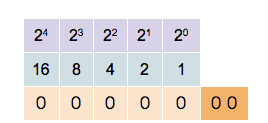0 0 0 1   decimal value 20 = 1
0 0 1 0   decimal value 21 = 2
0 1 0 0   decimal value 22 = 4
1 0 0 0   decimal value 23 = 8

The binary digits are called bits and in modern computers, the bits are grouped in units of 8. Each unit of 8 bits is called a byte and can contain values between 0 and 28 − 1 = 255. Of course, 255 is not a very large number and for most applications, larger numbers are needed. Most modern computer architectures support integers with 32 bits and 64 bits. Unsigned 32-bit integers range from 0 to 232 − 1 = 4, 294, 967, 295 ≈ 4.3 × 109 and unsigned 64-bit integers range from 0 to 264 − 1 = 18, 446, 744, 073, 709, 551, 615 ≈ 1.8 × 1019. It is worthwhile noting that many GPU architectures currently don’t natively support 64-bit numbers.

The computer’s processor contains registers that can store binary numbers. Thus a 64-bit processor contains 64-bit registers and has machine instructions that perform numerical operations on those registers. As an example, consider the addition operation. In binary, two numbers are added in much the same way as using long addition in decimal. Consider the addition of two 64 bit integers 7013356221863432502 + 884350303838366524. In binary, this is written as follows.

  01100001,01010100,01110010,01010011,01001111,01110010,00010001,00110110
+ 00001100,01000101,11010111,11101010,01110101,01001011,01101011,00111100
---------------------------------------------------------------------------
01101101,10011010,01001010,00111101,11000100,10111101,01111100,01110010


The process of adding two numbers is simple. From right to left, the digits of the two numbers are added. If the result is two or more, there will be a carry-over which is added to the next digit on the left.

You could add integers of any size using this prescription but, of course, in the computer numbers are limited by the number of bits they contain. Consider the following binary addition of (264 − 1) and 1 .

  11111111,11111111,11111111,11111111,11111111,11111111,11111111,11111111
+ 00000000,00000000,00000000,00000000,00000000,00000000,00000000,00000001
---------------------------------------------------------------------------
00000000,00000000,00000000,00000000,00000000,00000000,00000000,00000000


If you were dealing with mathematical integers, you would expect to see an extra digit 1 on the left. The computer cannot store that bit in the register containing the result but stores the extra bit in a special carry flag. In many computer languages, this unintentional overflow will go undetected and the programmer has to take care that numerical operations do not lead to unintended results.

### Signed Integers

The example above shows that adding two non-zero numbers can result in 0. This can be exploited to define negative numbers. In general, given a number a, the negative  − a is defined as the number that solves the equation
a + ( − a) = 0.
Mathematically, the N-bit integers can be seen as the group of integers modulo 2N. This means that for any number a ∈ {0, …, 2N − 1} the number  − a can be defined as
− a = 2N − a ∈ {0, …, 2N − 1}.
By convention, all numbers whose highest value binary bit is zero are considered positive. Those numbers whose highest value bit is one are considered negative. This makes the addition and subtraction of signed integers straightforward as the processor does not need to implement different algorithms for positive or negative numbers. Signed 32-bit integers range from  − 2, 147, 483, 648 to 2, 147, 483, 647, and 64-bit integers range from  − 9, 223, 372, 036, 854, 775, 808 to 9, 223, 372, 036, 854, 775, 807.

This format of storing negative numbers is called the two’s complement format. The reason for this name becomes obvious when observing how to transform a positive number to its negative.

01100001,01010100,01110010,01010011,01001111,01110010,00010001,00110110 (7013356221863432502)
10011110,10101011,10001101,10101100,10110000,10001101,11101110,11001010 (-7013356221863432502)


To invert a number, first, invert all its bits and then add 1. This simple rule of taking the two’s complement can be easily implemented in the processor’s hardware. Because of the simplicity of this prescription, and the fact that adding a negative number follows the same algorithm as adding a positive number, two’s complement is de-facto the only format used to store negative integers on modern processors.

### Exercises

1. Show that taking the two’s complement of an N-bit number a does indeed result in the negative  − a if the addition of two numbers is defined as the addition modulo 2N.
2. Find out how integers are represented in the programming language of your choice. Does this directly reflect the representation of the underlying architecture? I will be writing another post about this topic soon.
3. Most processors have native commands for multiplying two integers. The result of multiplying the numbers in two N-bit registers are stored in two N-bit result registers representing the high and low bits of the result. Show that the resulting 2N bits will always be enough to store the result.
4. Show how the multiplication of two numbers can be implemented using only the bit-shift operator and conditional addition based on the bit that has been shifted out of the register. The bit-shift operator simply shifts all bits of a register to the left or right.

#### String Art and Heart Curves

In my childhood, my parents would take us to the seaside for the summer holidays. One thing, other than the sun and the beach, that I distinctly remember were the nautic decorations in the cafes. One particular image was an abstract picture of a sailboat made from some nails hammered into a wooden board and pieces of string that were tied around those nails. It always fascinated me, how this simple arrangement of straight lines could create such a beautiful and dynamic image. Around the same time, in primary school, we used a similar technique in art class, with needle and thread and a piece of cardboard, to create an image of a snowflake.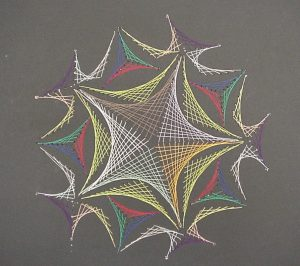It wasn’t until much later that I understood that the curves created by the thread could be described using mathematics. But while I knew that this had to do with maths somehow, during my school years I always thought that the maths was much too complicated for me to understand. Now, I have children and they have been doing similar art projects. From a teaching perspective, I am aware that these projects are intended to create an interest in maths. I think there is a missed opportunity when these line patterns are only used in primary school art lessons. The mathematics of the curves created by these lines can be accessible to secondary school students.

As an example, I want to present here the curve created by drawing straight lines in a circle. Here is the recipe:

• Draw a large circle on a piece of paper
• Draw a reasonably large number of equally spaced points around the circumference, about 20 to 30 should be OK
• Choose a point as point 0 and number the points around the circle, clockwise or anticlockwise
• Now draw a line
• from point 1 to point 2
• from point 2 to point 4
• from point 3 to point 6
• and so on, always connecting point $n$ with point $2n$.

Once the starting point has reached the opposite side, the endpoint will have reached point 0. Keep going by always incrementing the endpoint by two while moving the starting point by one.

You should end up with something like this.As you can see the lines you have drawn create an interesting pattern. In fact, the lines trace out a curve called the cardioid or heart curve. Each line you have drawn is a tangent to the cardioid. This means that each of the lines touches the cardioid in a single point.

Let’s take a look at this cardioid curve. It is a special case of an epicycloid. This family of curves is generated by tracing a point on the circumference of one circle as it rolls around another circle. In the case of the cardioid, both circles have the same radius. The point that traces the curve is chosen to lie on the circumference of the outer circle. In the picture below you can see how the cardioid is created.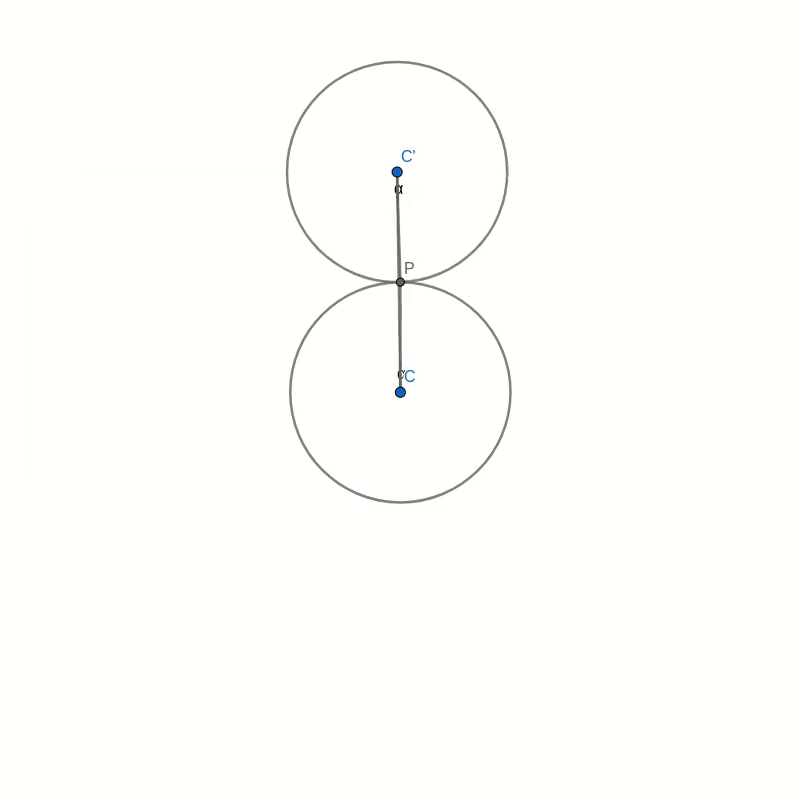The curves in the two pictures look similar and if you were to place one image on top of the other, you would not see any difference in the curves. That seems to suggest that the two are in fact identical. But comparing images is not a mathematical proof. And surely you don’t believe this statement just because everybody on the internet claims it to be true.

If you look around, you can find several proofs that show you that the curve created by our string art is indeed the cardioid. But most of these proofs make use of trigonometry. This makes them inaccessible to anybody who is not yet comfortable with trigonometric identities. I believe that a purely geometric proof is easier to understand and also more beautiful than resorting to sine and cosine functions.

## The proof

I order to prove the identity of the two curves we need to show two things. First, we need to show that our line construction intersects the cardioid in at least one point and we need to find that point. Then we need to show that the point that traces the cardioid moves in the direction of the line segment. This second part of the proof shows that the line segments are tangential to the cardioid.

### Part 1: Constructing a point on the cardioid

Look at the figure below. Don’t get too confused with all the lines and angles in the picture. I will explain everything bit by bit. Remember when you were drawing the string art picture. You were drawing a line from some start point to some endpoint. In the diagram below, I have taken one of those lines and named the start point B and the endpoint B’. I have also connected the origin point 0 to the start point B. Now, B’ moves around the circle twice as fast as point B. This means that the distance from B to B’ is the same as the distance from 0 to B. In other words, if you draw a line from the centre C of the big circle to B, the figure is symmetric with respect to that line.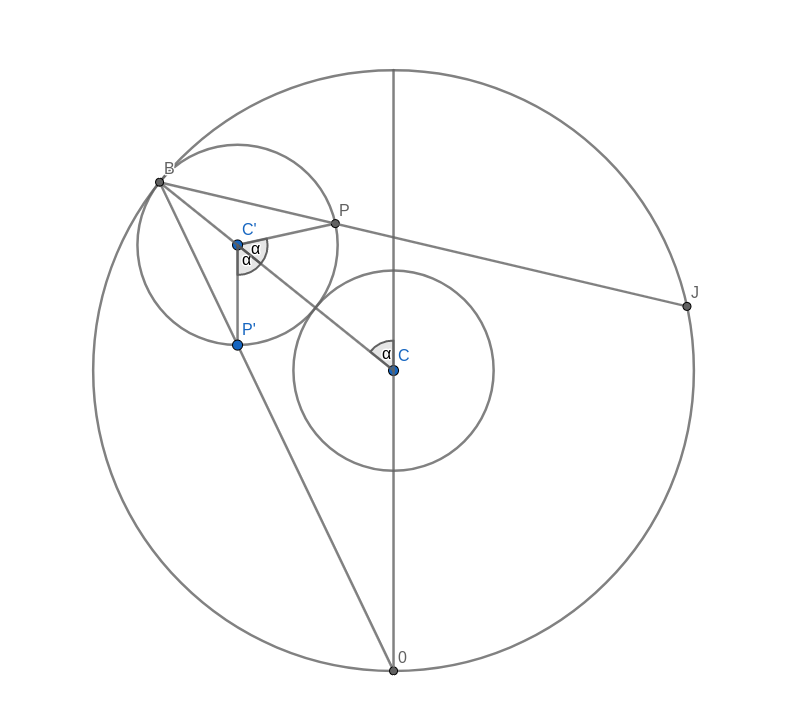Now, look at the two small circles. The circle at the centre C of the large circle stays fixed. The small circle centred at C’ on the connection between C and B is the circle that rolls along the circumference and creates the cardioid. The line B-B’ intersects this outer circle at a point P. We need to show that this intersection point P is the same as the point that stays fixed on the outer circle as it rolls along. We can prove this by showing that the angle C-C’-P is the same as the angle that C-C’ makes with the vertical through the central point C.

Take the intersection between the line A-B and the rolling circle and call it P’. You can immediately see that the triangles A-B-C and P’-B-C’ are similar. This means that the line P’-C’ is vertical, just like the line A-C. It follows that the angle C-C’-P’ is the same as the angle that C-C’ makes with the vertical because they are opposite angles. But because of the symmetry of the construction, this angle is also the same as C-C’-P.

With this, we have shown that the intersection point P is, in fact, a point on the cardioid curve.

### Part 2: Direction of motion of P

The motion of the point P, as the outer small circle rolls around the inner circle, is made up of two contributions. The first is the motion of the centre C’ orbiting around the inner circle. The second is the motion due to the rolling circle spinning around its own centre. We need to look at the direction and magnitude of each of these velocities.
Let’s say that the radius of the small circles is 1 and the angular velocity of the rotation of the outer circle around the inner is also 1. The exact values are not important because we only need to compare the two contributions of the velocity of point P. We don’t need to find its absolute value.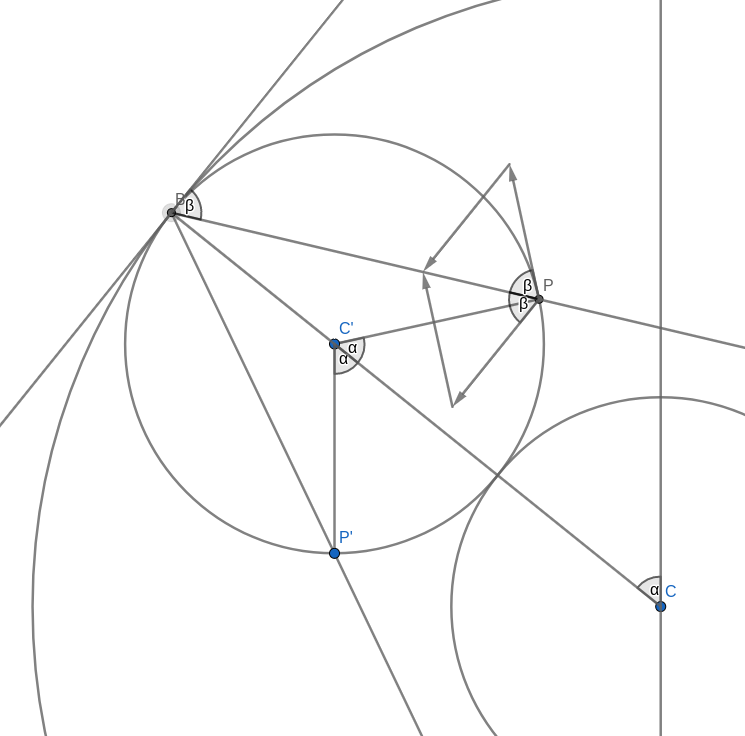Orbital Velocity

The orbital velocity is oriented perpendicular to the connection C-B which also is parallel to the tangent to the circle through B. This means that naturally, the angle between this velocity and the line B-P is the same as the angle between the tangent and B-P. To compare the magnitudes we have to remember some equation from physics. The speed of a point rotating around a centre is the product of the distance to the centre and the angular velocity. Let’s call the radius of the small circle $R$ and the angular velocity of the rotation of the outer circle around the inner circle $\omega$ (that’s the lowercase Greek letter omega). The distance of the outer centre to the centre of rotation is $2R$ and this makes the speed of the orbital rotation equal to
$$v_{\text{orbit}} = 2R\omega.$$

Now let’s look at the velocity of the spin rotation. The point P rotates around the centre of rotation C’. The radius is $R$ but the angular velocity is $2\omega$. To see this, look at the vertical line C’-P’. This line stays vertical at all times. In part 1 of the proof we already saw that that the angle C-C’-P’ is the same as the angle that C-C’ makes with the vertical, so as C’ moves around C by some angle, C-C’-P’ will increase by the same amount. But so will C-C’-P. This means that the angle P’-C-P increases twice as fast as the orbital angle. The resulting speed of the spin motion is
$$v_{\text{spin}} = R\times 2\omega.$$
We can see that the magnitude of the velocity of the spin motion is equal to that of the orbital motion. We also saw that the line B-P halves the angle between those two velocities. This means that the sum of these two velocities must lie in the direction of B-P. In other words, B-P is a tangent to the motion of the point P which is what we needed to prove.

## Summary

I admit that this proof is somewhat lengthy but I do think that it gives some interesting insight into why the pattern of lines created by our initial construction generates the cardioid curve. All the figures for this post were created with Geogebra and are available online.

#### Beware of square roots and exponentials!

In the last post I asked you the question, what is wrong with the following proof.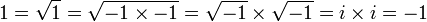As some of you noticed, the answer lies in the fact that the identity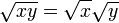is only true for positive real numbers x and y. In general one has to be careful with the identity

(xy)a = xaya

for non-integer a and non-positive x and y.

I posted this question because, although it ought to be, it is not always taught to students when complex numbers are introduced. Once students are taught complex numbers they feel that, now that they are free to take the square root of any real number, they don’t have to pay attention anymore to what they are doing. They apply the rules blindly, resting in false comfort that everything is now fine.

Another pitfall with complex numbers, that even educated people are not always aware of, is the fact that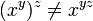if y and z are complex numbers, even for positive, real x. If it were we, could prove the following (here I assume that you are familiar with the complex exponential function).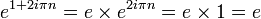This makes

(e1 + 2iπn)2iπn = (e)2iπn = 1

But, if the above identity were true, the last line would be equal to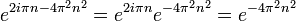Which, in general, is a positive real number not equal to 1.

#### Another proof that -1=1

Here is a little maths teaser for you. Since I was a student, I always loved those “proofs” that zero equals one. Of course, most of the time, this was achieved by sneakily dividing by zero somewhere along the way.

But yesterday I came across a proof that used a different, slightly more subtle trick and uses complex numbers. I apologise to any reader not familiar with complex numbers. Anyone interested can find a quick introduction here.

Enough introduction, here is the “proof”:Looks OK, but it can’t be right of course. So where is the error in this equation? Can you find out?

Update:
You can find the answer here.